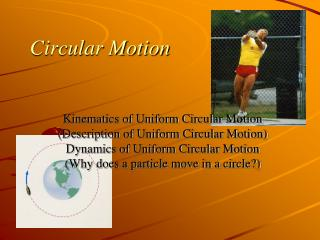Download PresentationCircular Motion

Circular Motion - PowerPoint PPT Presentation

Circular Motion. Kinematics of Uniform Circular Motion (Description of Uniform Circular Motion) Dynamics of Uniform Circular Motion (Why does a particle move in a circle?). Reading Question. Reviewing for the exam I have spent. Zero hours ½ hour 1 hour 1 ½ hours 2 hours 2 ½ hoursI am the owner, or an agent authorized to act on behalf of the owner, of the copyrighted work described.
Download PresentationCircular Motion

Download Policy: Content on the Website is provided to you AS IS for your information and personal use and may not be sold / licensed / shared on other websites without getting consent from its author.While downloading, if for some reason you are not able to download a presentation, the publisher may have deleted the file from their server.

- - - - - - - - - - - - - - - - - - - - - - - - - - E N D - - - - - - - - - - - - - - - - - - - - - - - - - -
Presentation Transcript
1. Circular Motion Kinematics of Uniform Circular Motion (Description of Uniform Circular Motion) Dynamics of Uniform Circular Motion (Why does a particle move in a circle?)

2. Reading Question Reviewing for the exam I have spent • Zero hours • ½ hour • 1 hour • 1 ½ hours • 2 hours • 2 ½ hours • 3 or more hours

3. Reading Question Circular motion is best analyzed in a coordinate system with 1. x- and y-axes. 2. x-, y-, and z-axes. 3. x- and z-axes. 4. r-, t-, and z-axes.

4. Reading Question Circular motion is best analyzed in a coordinate system with 1. x- and y-axes. 2. x-, y-, and z-axes. 3. x- and z-axes. 4. r-, t-, and z-axes.

5. Reading Question The quantity with the symbol w is called 1. the circular weight. 2. the angular velocity. 3. the circular velocity. 4. the centripetal acceleration.

6. Reading Question The quantity with the symbol w is called 1. the circular weight. 2. the angular velocity. 3. the circular velocity. 4. the centripetal acceleration.

7. Reading Question For uniform circular motion, the net force 1. points toward the center of the circle. 2. points toward the outside of the circle. 3. is tangent to the circle. 4. is zero.

8. Reading Question For uniform circular motion, the net force 1. points toward the center of the circle. 2. points toward the outside of the circle. 3. is tangent to the circle. 4. is zero.

9. Circular Motion Uniform circular motion is a particle moving at constant speed in a circle.

10. Circular Motion Is the speed changing? Is the velocity changing? Yes, changing in direction but not in magnitude. The period is defined as the time to make one complete revolution

11. s is arc length. r is the radius of the circle. Circular Motion How do we describe the position of the particle? The angle q is the angular position. Again q is defined to be positive in the counter-clock-wise direction. Angles are usually measured in radians.

12. Circular Motion Radians For a full circle.

13. The angular displacement is Average angular velocity Instantaneous angular velocity Circular Motion Angular velocity We will worry about the direction later. Like one dimensional motion +- will do. Positive angular velocity is counter-clock=wise.

14. Circular Motion Coordinate System

15. Circular Motion So, is there an acceleration?

16. Circular Motion So, is there an acceleration?

17. Student Workbook

18. Student Workbook

19. Student Workbook

20. Student Workbook

21. Student Workbook side of plane Which way is the plane turning? To the left

22. Yes directed toward the center of curvature (center of circle) Circular Motion So, is there an acceleration?

23. Class Questions A particle moves cw around a circle at constant speed for 2.0 s. It then reverses direction and moves ccw at half the original speed until it has traveled through the same angle. Which is the particle’s angle-versus-time graph? 1. 2. 3. 4.

24. Class Questions A particle moves cw around a circle at constant speed for 2.0 s. It then reverses direction and moves ccw at half the original speed until it has traveled through the same angle. Which is the particle’s angle-versus-time graph? 1. 2. 3. 4.

25. Class Questions Rank in order, from largest to smallest, the centripetal accelerations (ar)ato (ar)e of particles a to e. 1. 2. 3. 4. 5. 1. (ar)b> (ar)e> (ar)a > (ar)d> (ar)c 2. (ar)b= (ar)e> (ar)a = (ar)c> (ar)d 3. (ar)b> (ar)a= (ar)c = (ar)e> (ar)d 4. (ar)b> (ar)a= (ar)a > (ar)e> (ar)d 5. (ar)b> (ar)e> (ar)a = (ar)c> (ar)d

26. Class Questions Rank in order, from largest to smallest, the centripetal accelerations (ar)ato (ar)e of particles a to e. 1. 2. 3. 4. 5. 1. (ar)b> (ar)e> (ar)a > (ar)d> (ar)c 2. (ar)b= (ar)e> (ar)a = (ar)c> (ar)d 3. (ar)b> (ar)a= (ar)c = (ar)e> (ar)d 4. (ar)b> (ar)a= (ar)a > (ar)e> (ar)d 5. (ar)b> (ar)e> (ar)a = (ar)c> (ar)d

27. Circular Motion

28. Circular Motion • PROBLEM-SOLVING STRATEGY 7.1 Circular motion problems • MODEL Make simplifying assumptions. • VISUALIZE Pictorial representation. Establish a coordinate system with the r-axis pointing toward the center of the circle. Show important points in the motion on a sketch. Define symbols and identify what the problem is trying to find. • Physical representation. Identify the forces and show them on a free-body diagram. • SOLVE Newton’s second law is • . Determine the force components from the free-body diagram. Be careful with signs. • . SOLVE for the acceleration, then use kinematics to find velocities and positions. • ASSESS Check that your result has the correct units, is reasonable, and answers the questions.